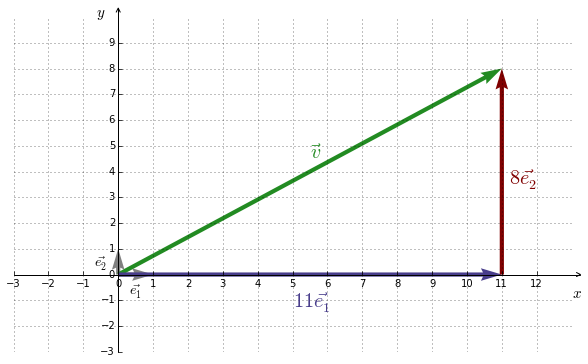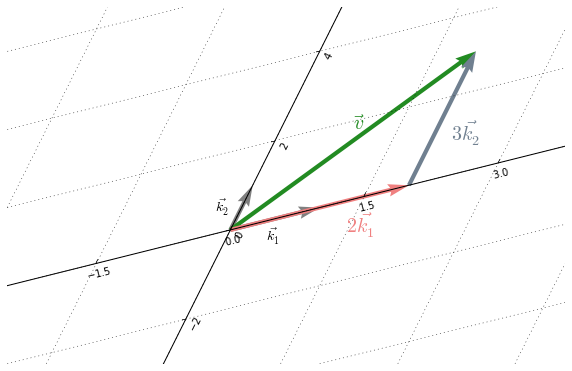## 1. Analogy

Generally speaking, a base is a reference system in which things are expressed. For example, the number $64$ is written 1000 in the base $2$ (binary base). They are in fact the same number but they are expressed (represented) in different bases :

The natural base for numbers is the base 10. As regards vectors, the natural base is called the standard basis (also called canonical basis or normal basis).

## 2. Representations

As for numbers, vectors are represented with respect to a basis.

#### Example I

Figure 8.1 shows the vector $\vec{v}= \left( \begin{smallmatrix} 11 \\ 8 \end{smallmatrix} \right)$ represented in the standard basis of $\mathbb{R}^2$, namely $STD = \{ \underbrace{\left( \begin{smallmatrix} 1 \\ 0 \end{smallmatrix} \right)}_\vec{e_1}, \underbrace{\left( \begin{smallmatrix} 0 \\ 1 \end{smallmatrix} \right)}_\vec{e_2} \}$.

In fact, $\vec{v}$ is the result of a linear combination of the basis vectors :Figure 8.1 : $\vec{v}$ in the standard basis of $\mathbb{R}^2$Figure 8.2 : $\vec{v}$ in a given basis

Let $B= \{ \underbrace{ \left( \begin{smallmatrix} 4 \\ 1 \end{smallmatrix} \right)}_{\vec{k_1}} \underbrace{ \left( \begin{smallmatrix} 1 \\ 2 \end{smallmatrix} \right) }_{\vec{k_2}} \}$ be a basis of $\mathbb{R}^2$. Let’s represent $\vec{v}$ in $B$.

For doing that, we need to solve the following equation :

In $(3)$ the coefficients $\lambda_i$ are the so-called components (also called coordinates). By solving $(3)$ we get $2$ and $3$ as components of $\vec{v}$ with respect to $B$.

Figure 8.2 shows $\vec{v}$ in $B$.

We have thus the vector $\vec{v}$ represented in two different basis :Figure 8.3 : $\vec{v}$ in two different basis

## 3. Conditions

For being a basis the following conditions have to be met :

• Any vector of a given space must be constructed from a linear combination of the basis vectors. That is the goal of a basis.
• The vectors of the basis must be linearly independent.

## 4. Change of basis

To convert a vector from a given basis $B$ into the standard basis, the following formula can be used :

#### Example II

Let’s convert $\vec{v}=\left( \begin{smallmatrix} 2 \\ 3 \end{smallmatrix} \right)$ from the basis $B=\{\left( \begin{smallmatrix} 4 \\ 1 \end{smallmatrix} \right),\left( \begin{smallmatrix} 1 \\ 2 \end{smallmatrix} \right)\}$, into the standard basis.

## Recapitulation

Any vector is expressed with respect to a given basis. By default, vectors are expressed in the standard basis.

The standard basis of the space $\mathbb{R}^{2}$ is $\{ \left( \begin{smallmatrix} 1 \\ 0 \end{smallmatrix} \right), \left( \begin{smallmatrix} 0 \\ 1 \end{smallmatrix} \right) \}$. The standard basis of the space $\mathbb{R}^{3}$ is $\{ \left( \begin{smallmatrix} 1 \\ 0 \\ 0 \end{smallmatrix} \right), \left( \begin{smallmatrix} 0 \\ 1 \\ 0 \end{smallmatrix} \right), \left( \begin{smallmatrix} 0 \\ 0 \\ 1 \end{smallmatrix} \right) \}$.

A basis of a given space generates, by linear combination, any vector of that space.

Are said to be the components (or coordinates) of a vector, the representation of a vector in a given basis.

All bases of $\mathbb{R}^{2}$ have $2$ vectors, all bases of $\mathbb{R}^{3}$ have $3$ vectors, etc. Generally speaking, all bases of $\mathbb{R}^{n}$ have $n$ vectors.